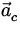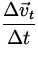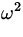# Define centripetal acceleration. Derive an expression for the centripetal acceleration of a particle moving with uniform speed v along a circular path of radius r.discuss the direction of this acceleration

Centripetal acceleration:

If an object moves in a circle it changes its velocity i.e. it is accelerating. This acceleration is known as the centripetal acceleration.

or we can say that the centripetal acceleration is defined as the rate of change of tangential velocity.

Now we know that, the centripetal force required to keep a particle of mass ‘m’ revolving in a circular path of radius ‘r’ with a speed ‘v’ is,

F = mv 2 /r

Now, centripetal acceleration (a c ) is the radial acceleration due to the centripetal force.

Using,

a c = F/m

=> a c = [mv 2 /r]/m

=> a c = v 2 /r

The centripetal acceleration is directed along the radius in the circular motion.

• 262

Centripetal acceleration is the rate of change of tangential velocity:=• The direction of the centripital acceleration is always inwards along the radius vector of the circular motion.
• The magnitude of the centripetal acceleration is related to the tangential speed and angular velocity as follows:

 ac ==r.

• -61

Centripetal acceleration is the rate of change of tangential velocity:=• The direction of the centripital acceleration is always inwards along the radius vector of the circular motion.
• The magnitude of the centripetal acceleration is related to the tangential speed and angular velocity as follows:

 ac ==r.

• -26
What are you looking for?